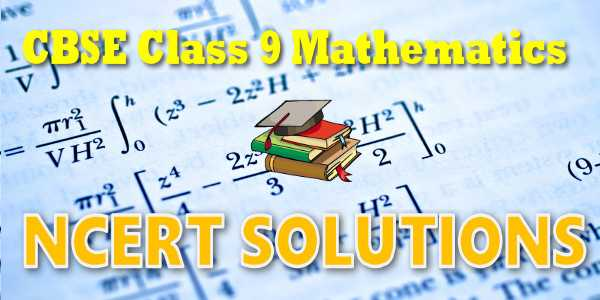No products in the cart.

# NCERT Solutions for Mathematics Linear Equations in Two Variables

## NCERT Solutions for Mathematics Linear Equations in Two Variables### Student Subscription

##### Unlock the exclusive content designed for the toppers

We will update content for this category shortly. Please visit this category after few days or subscribe to our newsletter by email for latest updates

### NCERT Solutions Chapter 04: Linear equation in two variables

In earlier classes, you have studied linear equations in one variable. Can you write down a linear equation in one variable? You may say that x+1=0, x+{tex}\sqrt 2 {/tex}=0 and {tex}\sqrt 2 y + \sqrt 3 - 0{/tex} are examples of linear equations in one variable. You also know that such equations have a unique (i.e., one and only one) solution. You may also remember how to represent the solution on a number line. In this chapter, the knowledge of linear equations in one variable shall be recalled and extended to that of two variables. You will be considered questions like: Does a linear equation in two variables have a solution? If yes, is it unique? What does the solution look like on the Cartesian plane? You shall also use the concepts you studied in Chapter 3 to answer these questions.

## NCERT Solutions for Class 9## myCBSEguide

Trusted by 1 Crore+ Students

#### Question Paper Creator

• Create papers in minutes
• Print with your name & Logo
• 5 Lakhs+ Questions
• Solutions Included
• Based on CBSE Syllabus
• Best fit for Schools & Tutors## Test Generator

Create papers at ₹10/- per paper## CUET Mock Tests

75,000+ questions to practice only on myCBSEguide app